The flips flops designed using logic gates are used in computers and other types of communication systems.

Basically they can store information, count operations, act like temporary memory

• Flip flops connected together forms a register.
• The registers capable of counting the clock pulses is a counter.

## Registers

A single flip flop can store a 1 bit word. Thus by connecting n flip flops  we can store n bit information.The stored information can be moved within the registers, upon application of pulses.These type of registers are known as shift registers. (For simplicity the flip flops considered here are all D flip flops.)
Where are these registers used?
• Serial In Serial Out shift register can be used for introducing time delay.
• Serial In Parallel Out shift register used for converting serial form of data to parallel form.
• Parallel In Serial Out shift register used to convert data from parallel to serial form.
• Also these shift registers can be used as Shift register counters where the output of the last stage is connected back to the serial input. This type of counter can produce specified sequences as output and is known as ring counter.
The applications mentioned above have classified the shift registers into 5 types. Let us look into it in detail.
1. Serial In Serial Out
As the name suggests the input to this register is fed serially and the output is also received serially.Either the data can be shifted left or towards right.
Illustrating for shifting left.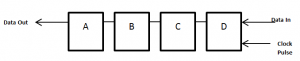Initially the output of all the flip flops are cleared to ‘0 ‘QaQbQcQd=0000. Consider the data we would like to enter as ‘1101’. This data is entered bit by bit. As the shift is towards left each bit gets shifted left.Thus the  output after the third clock pulse would be QaQbQcQd =0110. We can see that the data has been shifted left.Similarly the data can also be shifted right.
2. Serial In Parallel Out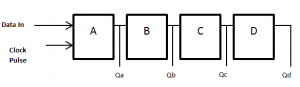Here the data input is given serially but the output can be obtained in parallel.After the input of data through the application of clock pulse in SISO only the last bit will be available as output at a time, but here all the bits are available as output at their respective output line simultaneously after shifting.
3. Parallel In Serial Out
The data can be loaded at any stage and the output be received serially by shifting the data. The diagram shown here is Parallel In Serial Out with shifting towards right. By applying clock pulse the loaded data is shifted towards right and received as output.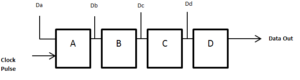4. Parallel In Parallel Out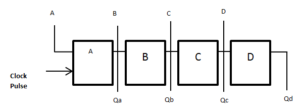This type would have more number of i/p and o/p pins when compared to other types of shift register.Data can be loaded at any stage and output can be taken from any output line after shifting towards right.
5. Ring Counter
Ring counter is formed by connecting the output of the first stage to the input of the next stage. The output of the last stage is connected as input to the first stage. The diagram below is a 4 bit ring counter.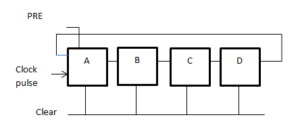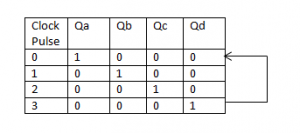The first flip flop is pre loaded with 1(Qa=1) and rest are cleared to 0.

On applying the first clock pulse the second stage is made 1 and rest 0. This 1 is retained and is just shifted around in the ring.

## Counters

Counters are registers which are able to count the clock pulses. These are broadly classified in to two types based on the clock pulse input,as Synchronous counter and Asynchronous counter.
1. Asynchronous Counter
This is also known as ripple counter. This is formed by connecting complementing flip flops together. The first flip flop is connected to the clock pulse and the rest of them are connected to output of the previous flip flop. Complementing flip flops are created using JK flip flops by tying their inputs together.
The diagram below is of 2 bit ripple up counter.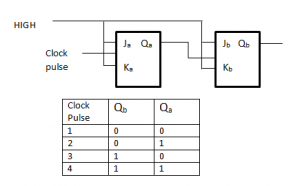The Clock input is for the first stage alone. The second stage is triggered by the output of the first stage.Due to propagation delay present in the flip flop, transition of input clock pulse and that of Qa output will never occur at simultaneously.This is known as asynchronous counter operation. Since both the inputs are tied to HIGH, for negative edge of the clock pulse output will toggle.
Ripple down counter can also be created by connecting the complemented output(Qa’) as clock input of next flip flop. This will count from maximum to zero.
Modulo- n- counter
Ripple counters can be modified to act as modulo- n- counters with the help of Nand gates. The flip flops are reset when the output of the Nand gate goes low. Consider MOD 5 counter. The counter should be reset when the state 101 is reached. A Nand gate can be added to the output of C and A stage.When the output of both the stage reaches 1 the Nand gate resets all flip flops to 0 and once again it can be used to count until 5.Have a look at the counter design and the state sequence given below for MOD 5 counter.
2. Synchronous Counter
All the flip flops are clocked to the same counter and will be triggered at the same time. This type of counter is known as simultaneous counter.
The diagram below is of two stage synchronous counter. Both are connected to the same clock signal and the output of the first flip flop acts as the input to the next flip flop.The operation of the counter would be as follows
In the initial state the outputs of the flip flops are assumed to be 0. Qa=Qb=0. On application of the first clock pulse flip flop A will toggle as both the inputs are high thus the output will be Qa=1 and Qb=0. On second clock pulse both the flip flops will toggle(both inputs being high for both the flip flops) resulting in the output Qa=0 and Qb=1.On the third clock pulse only A will toggle as the input to B flip flop is 0. On fourth clock pulse both A and B will toggle resulting in the counter recycled to its original state.Have a look at the state sequence diagram. After the fourth clock pulse the counter is reset to Qa=0 and Qb=0.
In this type of counter every stage is dependent on the output of previous stage except the first stage where the input is given as HIGH at all times. Some modifications are required with the circuit for smooth operation as the stages increase. For example in a 3 stage synchronous counter we need to add AND gate to the output of stage A and B so that stage C will change only when the output of stage A and B are 1. At all other times the input of stage C will be held at low and will not change state.Similar modifications are done as stages increase.
Though the logic circuit involves complex design as the stages increase this type is preferred over ripple counters as there is no propagation delay.
Applications of Counter
Used as
• Digital clocks
• Frequency counter
• Binary Counter etc.
Share.